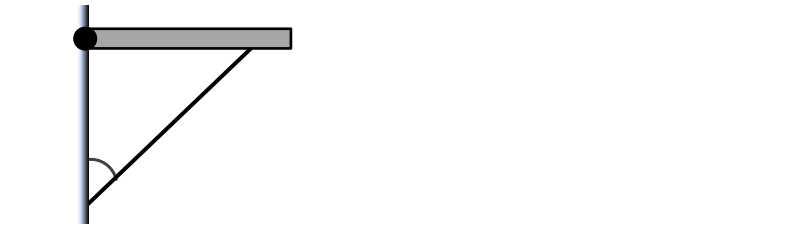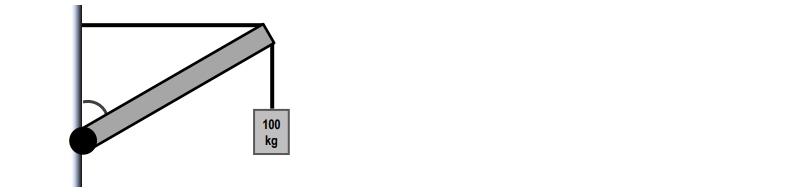Ch 15: Rotational EquilibriumWorksheetSee all chapters
 Ch 01: Intro to Physics; Units 1hr & 22mins 0% complete Worksheet Ch 02: 1D Motion / Kinematics 4hrs & 13mins 0% complete Worksheet Ch 03: Vectors 2hrs & 2mins 0% complete Worksheet Ch 04: 2D Kinematics 2hrs 0% complete Worksheet Ch 05: Projectile Motion 2hrs & 57mins 0% complete Worksheet Ch 06: Intro to Forces (Dynamics) 3hrs & 42mins 0% complete Worksheet Ch 07: Friction, Inclines, Systems 4hrs & 32mins 0% complete Worksheet Ch 08: Centripetal Forces & Gravitation 3hrs & 51mins 0% complete Worksheet Ch 09: Work & Energy 3hrs & 56mins 0% complete Worksheet Ch 10: Conservation of Energy 7hrs & 4mins 0% complete Worksheet Ch 11: Momentum & Impulse 5hrs & 35mins 0% complete Worksheet Ch 12: Rotational Kinematics 3hrs & 4mins 0% complete Worksheet Ch 13: Rotational Inertia & Energy 7hrs & 7mins 0% complete Worksheet Ch 14: Torque & Rotational Dynamics 2hrs & 9mins 0% complete Worksheet Ch 15: Rotational Equilibrium 4hrs & 10mins 0% complete Worksheet Ch 16: Angular Momentum 3hrs & 6mins 0% complete Worksheet Ch 17: Periodic Motion 2hrs & 17mins 0% complete Worksheet Ch 19: Waves & Sound 3hrs & 25mins 0% complete Worksheet Ch 20: Fluid Mechanics 4hrs & 39mins 0% complete Worksheet Ch 21: Heat and Temperature 4hrs & 9mins 0% complete Worksheet Ch 22: Kinetic Theory of Ideal Gasses 1hr & 40mins 0% complete Worksheet Ch 23: The First Law of Thermodynamics 1hr & 49mins 0% complete Worksheet Ch 24: The Second Law of Thermodynamics 4hrs & 56mins 0% complete Worksheet Ch 25: Electric Force & Field; Gauss' Law 3hrs & 32mins 0% complete Worksheet Ch 26: Electric Potential 1hr & 55mins 0% complete Worksheet Ch 27: Capacitors & Dielectrics 2hrs & 2mins 0% complete Worksheet Ch 28: Resistors & DC Circuits 3hrs & 20mins 0% complete Worksheet Ch 29: Magnetic Fields and Forces 2hrs & 35mins 0% complete Worksheet Ch 30: Sources of Magnetic Field 2hrs & 30mins 0% complete Worksheet Ch 31: Induction and Inductance 3hrs & 38mins 0% complete Worksheet Ch 32: Alternating Current 2hrs & 37mins 0% complete Worksheet Ch 33: Electromagnetic Waves 1hr & 12mins 0% complete Worksheet Ch 34: Geometric Optics 3hrs 0% complete Worksheet Ch 35: Wave Optics 1hr & 15mins 0% complete Worksheet Ch 37: Special Relativity 2hrs & 10mins 0% complete Worksheet Ch 38: Particle-Wave Duality Not available yet Ch 39: Atomic Structure Not available yet Ch 40: Nuclear Physics Not available yet Ch 41: Quantum Mechanics Not available yet

# Beam / Shelf Against a Wall

See all sections

Concept #1: Beam / Shelf Against a Wall

Transcript

Practice: A beam 200 kg in mass and 6 m in length is held horizontally against a wall by a hinge on the wall and a light rod underneath it, as shown. The rod makes an angle of 30° with the wall and connects with the beam 1 m from its right edge. Calculate the angle that the Net Force of the hinge makes with the horizontal (use +/– for above/below +x axis).Example #1: Beam supporting an object

Transcript

Hey guys! Let's check out this example of a horizontal beam that's being held against the wall with the rope. It also has a 500-kg mass at its end here. The beam has mass of 400. We got the 500 there, so I'm going to call 400 little m and the 500 will be big M. The beam has a length l = 8 meters. I'm going to assume uniform mass distribution on the beam which means that the 400-kilograms acts right here. I have little mg will be 4000. I have big mg right here which is going to be 5000. This is a distance of half the length, 4 meters. This is going to be 4 meters as well. Notice how I have a tension here. IÕm going to actually delete that T there. Because of equilibrium on the 500, this tension here equals 5000 equals the mg. It's just a connecting force. You can just think that the bar is essentially being pulled right here with mg. IÕm going to do that so instead of having mg and T, I just have the same one force. The truth is if you had two mgÕs, little mg and big Mg, right here. IÕm also going to have a tension here. This tension, we're actually going to write it out. This tension gets split into Tx and Ty. This is going to be our Tx right here and this is going to be our Ty right here. The angle between the cable and the horizontal is 53, so this angle right here is of 53 degrees. It says it connects 2 meters from the right edge of the beam. This distance here is 2 meters, which means that this distance here will also be 2 meters. A 500-kilogram object hangs from the right edge of the beam. We see that. We wanna calculate the magnitude of the net force the hinge applies on the beam. What is H net? Remember, the hinge will apply an x-force and a y-force. What we're going to do is we're going to have an H force this way, Hx, to cancel out the Tx. Hy, we donÕt know if it's up or down so we're going to assume Hy is up. Let me write this down here. We're going to assume Hy to be up and let's see what we get. Once I find Hx and Hy, I'll be able to calculate H net which is this. H net will be the square root of Hx^2 + Hy^2. What we're really looking for in this problem is we're looking for Hx and Hy first so that we can find the magnitude of the net force here. We'll be able to continue that there. How are going to find Hx and Hy? Like every torque, every equilibrium problem, we're going to write that sum of all torques equals zero and the sum of all forces equals zero.